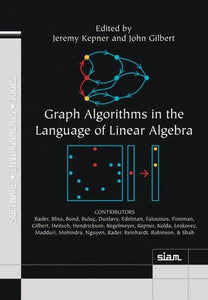Graph Algorithms In The Language Of Linear Algebra (Software, Environments, And Tools)

• Publish Date: 2011-07-14
• Binding: Hardcover
• Author: Jeremy Kepner;John Gilbert
Regular price \$151.21 Sale price \$147.19

Attention: For textbook, access codes and supplements are not guaranteed with used items.

Graphs are among the most important abstract data types in computer science, and the algorithms that operate on them are critical to modern life. Graphs have been shown to be powerful tools for modeling complex problems because of their simplicity and generality. Graph algorithms are one of the pillars of mathematics, informing research in such diverse areas as combinatorial optimization, complexity theory, and topology. Algorithms on graphs are applied in many ways in today s world - from Web rankings to metabolic networks, from finite element meshes to semantic graphs.

The current exponential growth in graph data has forced a shift to parallel computing for executing graph algorithms. Implementing parallel graph algorithms and achieving good parallel performance have proven difficult. This book addresses these challenges by exploiting the well-known duality between a canonical representation of graphs as abstract collections of vertices and edges and a sparse adjacency matrix representation. This linear algebraic approach is widely accessible to scientists and engineers who may not be formally trained in computer science. The authors show how to leverage existing parallel matrix computation techniques and the large amount of software infrastructure that exists for these computations to implement efficient and scalable parallel graph algorithms. The benefits of this approach are reduced algorithmic complexity, ease of implementation, and improved performance.

Graph Algorithms in the Language of Linear Algebra is the first book to cover graph algorithms accessible to engineers and scientists not trained in computer science but having a strong linear algebra background, enabling them to quickly understand and apply graph algorithms. It also covers array-based graph algorithms, showing readers how to express canonical graph algorithms using a highly elegant and efficient array notation and how to tap into the large range of tools and techniques that have been built for matrices and tensors; parallel array-based algorithms, demonstrating with examples how to easily implement parallel graph algorithms using array-based approaches, which enables readers to address much larger graph problems; and array-based theory for analyzing graphs, providing a template for using array-based constructs to develop new theoretical approaches for graph analysis.

Audience: This book is suitable as the primary text for a class on linear algebraic graph algorithms and as either the primary or supplemental text for a class on graph algorithms for engineers and scientists without training in computer science.

Contents: List of Figures; List of Tables; List of Algorithms; Preface; Acknowledgments; Part I: Algorithms: Chapter 1: Graphs and Matrices; Chapter 2: Linear Algebraic Notation and Definitions; Chapter 3: Connected Components and Minimum Paths; Chapter 4: Some Graph Algorithms in an Array-Based Language; Chapter 5: Fundamental Graph Algorithms; Chapter 6: Complex Graph Algorithms; Chapter 7: Multilinear Algebra for Analyzing Data with Multiple Linkages; Chapter 8: Subgraph Detection; Part II: Data: Chapter 9: Kronecker Graphs; Chapter 10: The Kronecker Theory of Power Law Graphs; Chapter 11: Visualizing Large Kronecker Graphs; Part III: Computation: Chapter 12: Large-Scale Network Analysis; Chapter 13: Implementing Sparse Matrices for Graph Algorithms; Chapter 14: New Ideas in Sparse Matrix-Matrix Multiplication; Chapter 15: Parallel Mapping of Sparse Computations; Chapter 16: Fundamental Questions in the Analysis of Large Graphs; Index.